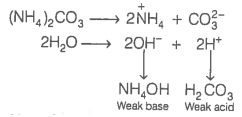48. Assertion (A) Aqueous solution of ammonium carbonate is basic.

Reason (R) Acidic/basic nature of a salt solution of a salt of weak acid and weak base depends on Ka and Kb value of the acid and the base forming it.

(a) Both A and R are true and R is the correct explanation of A

(b) Both A and R are true but R is not the correct explanation of A

(c) A is true but R is false

(d) Both A and R are false

(a) Both assertion and reason are true and reason is the correct explanation of assertion.If Kb of ${\mathrm{NH}}_{4}\mathrm{OH}>$Ka of ${\mathrm{H}}_{2}{\mathrm{CO}}_{3}$
The solution is basic.
or, If Ka of ${\mathrm{H}}_{2}{\mathrm{CO}}_{3}$>Kb of ${\mathrm{NH}}_{4}\mathrm{OH}$; the solution is acidic.